Figure 12.1 A base plot with no x-axis labels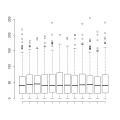R code
 Figure 12.2 Annotating a base plot with grid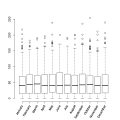R code
 Figure 12.3 Embedding a base plot within lattice output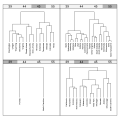R code
 Figure 12.4 A base barplot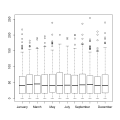R code
 Figure 12.5 A grid version of a base barplotR code
 Figure 12.6 Embedding a base plot with grid.echo()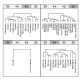R code
 Figure 12.7 Combining a base plot with a ggplot2 plot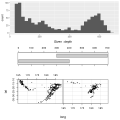R code